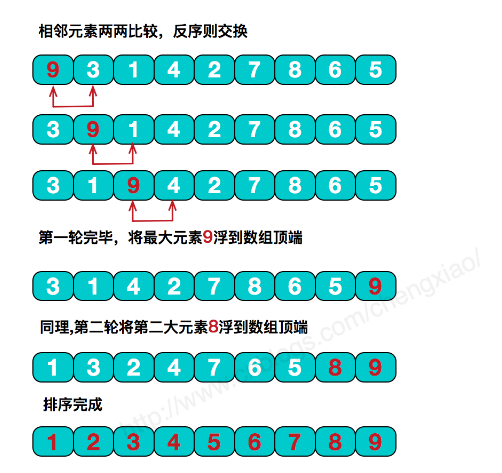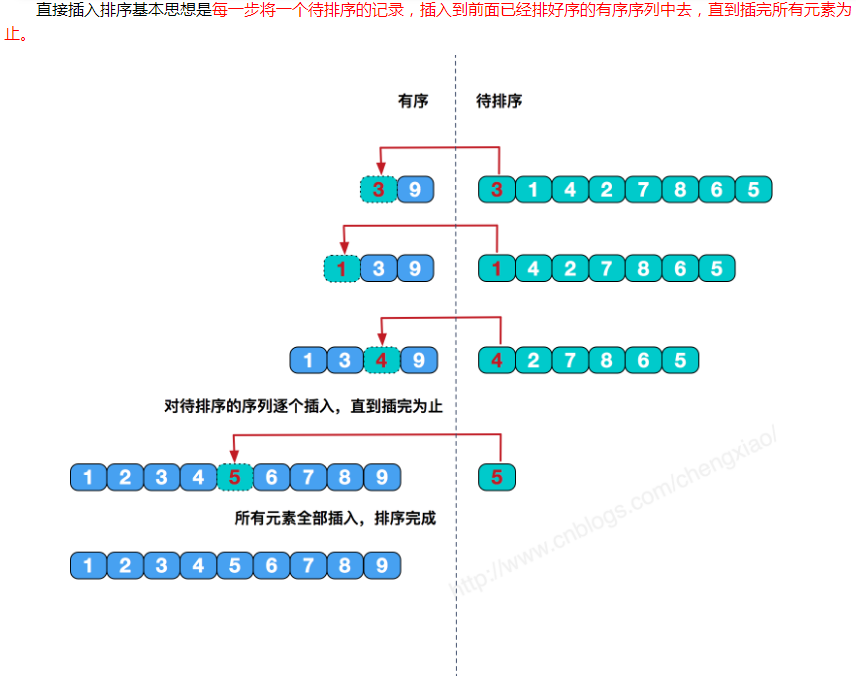• Java数组快速排序 1.快速排序的思想 ​ 通过一趟排序将待排记录分隔成独立的两部分，其中一部分记录的关键字均比另一部分的关键字小，则可分别对这两部分记录继续进行排序，以达到整个序列有序。 2.实现原理 取出...
Java数组快速排序
1.快速排序的思想
​ 通过一趟排序将待排记录分隔成独立的两部分，其中一部分记录的关键字均比另一部分的关键字小，则可分别对这两部分记录继续进行排序，以达到整个序列有序。
2.实现原理
取出数组的第一个元素存放到temp中,空出第一个元素位置从最后一个元素开始比较,找到比temp小的放置到第一个元素位置,空出新的位置
从第二个元素开始比较,找到比temp大的元素放到新空出的位置,
再从右边向左边寻找
…
一次遍历结束,将temp放入到空位置
递归完成排序
3.代码演示
public static void main(String[] args) {
int[] arr = {1, 2, 5, 2, 1, 6, 43, 8, 32, 4, 54, 6, 24, 32, 65, 4, 23};
sort(arr, 0, arr.length-1);
System.out.println(Arrays.toString(arr));
}
public static void sort(int[] array, int left, int right) {
if (left > right) {
return;
}
int i = left;
int j = right;
int temp = array[i];
while (i < j) {
//从右边开始遍历
while (temp <= array[j] && i < j) {
j--;
}
if (i < j) {
array[i] = array[j];
i++;
}
//从左边开始查找
while (temp >= array[i] && i < j) {
i++;
}
if (i < j) {
array[j] = array[i];
j--;
}
}
array[i] = temp;
sort(array, left, i - 1);
sort(array, i + 1, right);
}

展开全文算法
• end){//条件不满足，即快排完毕，结束递归 int index=getIndex(start,end,array);//先获取基准数坑位 doQuickSort(start,index-1,array);//递归调用 比基准数小的 doQuickSort(index+1,end,array);//递归调用 比...java
• //递归的对左侧序列进行快序排序 quicksort(arr,pt + 1,end);//递归的对右侧序列进行排序 }} public int ptr(int arr[],int first,int end){ int i = first;  int j = end; while( i ){ while...
package com.array.sort;

public class QuickSort {

/**

* @param args

*/

public static void main(String[] args) {

// TODO Auto-generated method stub

int arr [] = {12,43,11,5,23,76,99,56,22};

QuickSort a = new QuickSort();

a.printss(arr);

a.quicksort(arr, 0, arr.length-1);

System.out.println("\n after:");

a.printss(arr);

}

public void printss(int arr[]){

for(int i = 0;i< arr.length;i++){

System.out.print(arr[i] + ",");

}

}

public void quicksort(int arr[],int first,int end){

if(first < end){

int pt = ptr(arr, first, end);//问题分解，pt是轴值在序列中的位置

quicksort(arr,first,pt - 1);//递归的对左侧序列进行快序排序

quicksort(arr,pt + 1,end);//递归的对右侧序列进行排序

}}
public int ptr(int arr[],int first,int end){

int i = first;

int j = end;

while( i < j ){

while(i < j && arr[i] <= arr[j])

j--;

if(i < j)

{

int temp = arr[j];

arr[j] = arr[i];

arr[i] = temp;

i++;

}

while(i < j && arr[i] <= arr[j])

i++;

if(i < j)

{

int temp = arr[j];

arr[j]= arr[i];

arr[i] = temp;

j--;

}

}

return i;
}
}
展开全文• 我们对数组进行排序首先要了解一个类。Java.util.Arrays。  此类包含用来操作数组（比如排序和搜索）的各种方法。此类还包含一个允许将数组作为列表来查看的静态工厂。 除非特别注明，否则如果指定数组引用为 ...
       我们对数组进行排序首先要了解一个类。Java.util.Arrays。

此类包含用来操作数组（比如排序和搜索）的各种方法。此类还包含一个允许将数组作为列表来查看的静态工厂。
除非特别注明，否则如果指定数组引用为 null，则此类中的方法都会抛出 NullPointerException。 我们使用Arrays对数组进行排序。
Arrays.sort(数组)；
1、若数组是数字类型数组按升序排列；
2、若数组是char类型按自然顺序排列；
3、若数组是Object[]类型，根据元素的自然顺序对指定对象数组按升序进行排序。
例：输出结果:当然我们也可以指定数组的某一段进行排序比如我们要对数组下表0-2的部分(假设数组长度大于3)进行排序，其他部分保持不变，我们可以使用:
Arrays.sort(arrInt,0,3);
这样只对下标索引0-3排序,不会对后面的元素产生影响。
接下来，一一讲解数组中个排序算法的原理：冒泡排序、插入排序、选择排序、快速排序。
（1），冒泡排序
基本思想：在要排序的一组数中，对当前还未排好序的范围内的全部数，自上而下对相邻的两个数依次进行比较和调整，让较大的数往下沉，较小的往上冒。即：每当两相邻的数比较后发现它们的排序与排序要求相反时，就将它们互换。
public void upSort(int[] arrInt){
for(int i=0;i<arrInt.length-1;i++){   //冒泡排序 for(int j=0;j<arrInt.length-1;j++){ if(arr[j]>arr[j+1]){ int temp=arr[j]; arr[j]=arr[j+1]; arr[j+1]=temp; } } }
}
（2）插入排序
public void insortSort(int[] arr){

for(int i=1;i<arr.length;i++){

int k=a[i];

int j;

for(j=i-1;j>=0&&k<arr[j];j--){

a[j+1]=a[j];

}

a[j+1]=k;

}

}

(3)选择排序

基本思想：在要排序的一组数中，选出最小的一个数与第一个位置的数交换；
然后在剩下的数当中再找最小的与第二个位置的数交换，如此循环到倒数第二个数和最后一个数比较为止。

public void choiseSort(int[] arr)

for(int i=0;i<arr.length-1;i++){ for(int j=i+1;j<arr.length;j++){ if(arr[i]>arr[j]){ int t=arr[i]; arr[i]=arr[j]; arr[j]=t;   } } }

}

(4)快速排序

基本思想：选择一个基准元素,通常选择第一个元素或者最后一个元素,通过一趟扫描，将待排序列分成两部分,

一部分比基准元素小,一部分大于等于基准元素,此时基准元素在其排好序后的正确位置,然后再用同样的方法

递归地排序划分的两部分。

public class QuickSort {  public static int sort(int arr[],int left,int right){  int key = arr[left];  while(left<right){

// 左比较 while(left < right && arr[right] >= key){ right--; }  if(left<right && arr[right] < key){ arr[left] = arr[right]; }  // 右比较 while(left<right && arr[left] <= key){ left++; }

if(left<right && arr[left] > key){ arr[right] = arr[left]; }  } arr[right] = key; return left;  }

public static void getSort(int arr[],int left,int right){  if(left<right){ int index = sort(arr, left, right); getSort(arr,left,index-1); getSort(arr,index+1,right); } }


展开全文• Java数组排序：Java sort()方法 Arrays.sort(scores); Java数组排序：Java冒泡排序（Bubble Sort）法 System.out.println("通过冒泡排序方法对数组进行排序："); for(int i=0;i<score.length-1;i++) { //...
Java数组排序：Java sort()方法
Arrays.sort(scores);
Java数组排序：Java冒泡排序（Bubble Sort）法
System.out.println("通过冒泡排序方法对数组进行排序：");
for(int i=0;i<score.length-1;i++)
{
//比较相邻两个元素，较大的数往后冒泡
for (int j=0;j<score.length-1-i;j++)
{
if(score[j]>score[j+1])
{
double temp=score[j+1];    //把第一个元素值保存到临时变量中
score[j+1]=score[j];    //把第二个元素值转移到第一个元素变量中
score[j]=temp;    //把临时变量（第一个元素的原值）保存到第二个元素中
}
System.out.print(score[j]+ " ");    //对排序后的数组元素进行输出
}
}Java数组排序：Java快速排序（Quicksort）法
(1) 声明静态的 getMiddle() 方法，该方法需要返回一个 int 类型的参数值，在该方法中传入 3 个参数。代码如下：
public static int getMiddle(int[] list,int low,int high)
{
int tmp=list[low];    //数组的第一个值作为中轴（分界点或关键数据）
while(low<high)
{
while(low<high&&list[high]>tmp)
{
high--;
}
list[low]=list[high];    //比中轴小的记录移到低端
while(low<high&&list[low]<tmp)
{
low++;
}
list[high]=list[low];    //比中轴大的记录移到高端
}
list[low]=tmp;    //中轴记录到尾
return low;    //返回中轴的位置
}
(2) 创建静态的 unckSort() 方法，在该方法中判断 low 参数是否小于 high 参数，如果是则调用 getMiddle() 方法，将数组一分为二，并且调用自身的方法进行递归排序。代码如下：
public static void unckSort(int[] list,int low,int high)
{
if(low<high)
{
int middle=getMiddle(list,low,high);    //将list数组一分为二
unckSort(list,low,middle-1);    //对低字表进行递归排序
unckSort(list,middle+1,high);    //对高字表进行递归排序
}
}
(3) 声明静态的 quick() 方法，在该方法中判断传入的数组是否为空，如果不为空，则调用 unckSort() 方法进行排序。代码如下：
public static void quick(int[] str)
{
if(str.length>0)
{
//查看数组是否为空
unckSort(str,0,str.length-1);
}
}
Java数组排序：Java选择排序法
for(int i=1;i<number.length;i++)
{
index=0;
for(int j=1;j<=number.length-i;j++)
{
if(number[j]>number[index])
{
index=j;    //查找最大值
}
}
end=number[index]+" "+end;    //定位已排好的数组元素
int temp=number[number.length-i];
number[number.length-1]=number[index];
number[index]=temp;
System.out.print("【");
for(int j=0;j<number.length-i;j++)
{
System.out.print(number[j]+" ");
}
System.out.print("】"+end);
}
Java数组排序：Java直接插入法public static void main(String[] args)
{
int[] number={13,15,24,99,4,1};
System.out.println("排序前：");
for(int val:number)
{    //遍历数组元素
System.out.print(val+" ");    //输出数组元素
}
int temp,j;
for(int i=1;i<number.length;i++)
{
temp=number[i];
for(j=i-1;j>=0&&number[j]>temp;j--)
{
number[j+1]=number[j];
}
number[j+1]=temp;
}
System.out.println("\n排序后：");
for(int val:number)
{    //遍历数组元素
System.out.print(val+" ");    //输出数组元素
}
}

展开全文• 当你拿到一个无序的数组时，经过一次排序，将一个数组分成了两个数组，在经过递归 排序，就会得到一个排序数组。 **想法：**基本上会选择数组中的第一个元素作为一个比较的基准点，将所有的比基准点小的值放在它的...
• 排序思路：假设有n个数需要进行全排列,我们可以把每个数都放到第一个位置,然后剩下的n-1个数进行全排列。 * 即有n*(n-1)!种可能性,与n个数进行全排列的n!次可能性一致 利用递归的方式,依此类推当剩下的数个数为1...
• Java数组排序总结(冒泡_选择_插入_希尔)__递归算法的复杂度，实用算法
• Java 数组的快速排序 快速排序思想 快速排序的思想通过先选择一个基准点，然后通过左右两个指针先后遍历，并且依次比较数值与基准点的大小，比基准点大的数据放到右边，比基本点小的数据放到左右。在循环遍历左右...
• 将已知数组进行排序 代码如下： public class MethodDemo15 { public static void main(String[] args) { //创建数组 int[] arr = {9, 8, 7, 6, 5, 4, 3, 2, 1}; //排序 quickSort(arr, 0, arr.length - 1); ...
• 快速排序的基本思想是：通过一趟排序，将要排序的数据分隔成独立的两部分，其中一部分的所有数据比另外一部分的所有数据都要小，然后再按此方法对这两部分数据分别进行快速排序，整个排序过程可以递归进行，以此使...
• 如果空间已没有，便需要扩容.Java数组扩容采用建立一个新的数组，然后将原数据的数据拷贝过来。这里需要用到函数System.arraycopy。 public static void arraycopy(Object src, int srcPos, Object dest, int ...
• 3.合并数组 4.填充 5.删除数 6.字符串的输出 public class TestDemo1 { //插入元素 public static int[] Insert(int[] array,int val,int index) { int[] array2 = new int[array.leng...
• 提到数组，大家都不陌生，通常遇到数组会涉及到一些算法，像数组排序，找唯一，反转等，算法层出不穷。今天介绍一个使用递归算法进行对一个整型数组，判断数组中是否有副本。 public static boolean unique(int[] ...
• 数组快速排序递归实现 import java.text.SimpleDateFormat; import java.util.Arrays; import java.util.Date; public class testDemo { private static int[] arrs; /** * 快速排序 * @param args */ ...
• 排序 数→和→平均值 循环比较查找最大值最小值 二维数组模拟数据管理系统 收藏算法博客 获取运算前后时间比较长短 long t1 = System.currentTimeMillis(); 递归 不尚贤，使民不争；不贵难得之
•  * 利用递归实现数组排序  * @param arr 一个数组  * @param stop 这是一个参数，调用此方法时，默认写0  * @return 返回一个从小到大排列的数组  * 这个方法其内部实现原理：每个数都与第一个数比较，...算法
• 数组排序的实现方式很多，在这里我采用的是递归的方式，还是上面的例子：abcd (1) 首先将首字母分别与后面的n（n>=1）个字母交换 abcd bacd cbad dbca (1)将交换后的字符数组第n位和n+1位进行交换并...
• 一、排序 排序：将杂乱无章的数据元素，通过一定的方法按关键字顺序排列的过程叫做排序排序也是计算机内经常进行的一种操作，其目的是将一组“无序”的记录序列调整为“有序”的记录序列。
•内存管理
• 1.选择排序法 每一趟从待排序的数据元素中选出最小（或最大)的一个元素，顺序放在已排好序的数列最后，直到全部待排序的数据元素排完。 for (int i = 0; i ; i++) { int min = array[i]; for (int...class string
• 代码写的最规范的一次之一 /捂脸 ... * 递归地进行拆分数组并合并，如[1,2,3,4] 第一次拆分为[1,2],[3,4], 第二拆分为, , 然后合并 * * @param a 待排序数组 * @param beginIndex
• Java递归方法实现二分查找法 1.二分查找输入查找值，返回查找值的数组下标（查找的数组arr，数组的开头start，数组的结尾end，查找的值key） 先判断输入的start（开头）是否比end（结尾）大，如果比end（结尾）大...数据结构 算法 二分查找
• Java进阶课程代码包含文件读写IO流，递归算法思想，数组排序、生产模式（单体、工厂）...

# java数组递归排序java 订阅